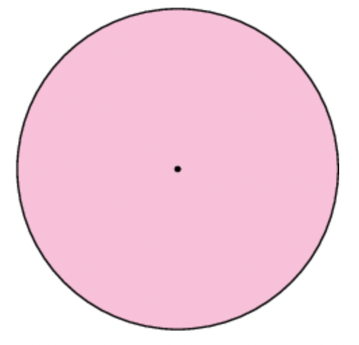Home Practice
For learners and parents For teachers and schools
Textbooks
Full catalogue
Pricing SupportLog in

We think you are located in United States. Is this correct?

# Using formulas

## 21.3 Using formulas

You have already used formulas to calculate the perimeter, area and volume of different objects and shapes. Sometimes those formulas need to be manipulated depending on what information is known and which value needs to be calculated. For example, if you are given the area of a rectangle and the measurement of one of its sides, you can calculate the measurement of the other side.

For example, let’s use the formula for the area of a rectangle $$A = l \times b$$ to make some calculations.

## Worked Example 21.4: Working with area of rectangle formula

Use the formula for the area of a rectangle to calculate the following:

• The area, if the length is $$45 \text{ mm}$$ and the width is $$25 \text{ mm}$$.
• The length, if the area is $$240 \text{ mm}^2$$ and the width is $$40 \text{ mm}$$.
• The width, if the area is $$144 \text{ cm}^2$$ and the length is $$12 \text{ cm}$$.

### Use the length and width to determine the area.

The length is $$45 \text{ mm}$$ and the width is $$25 \text{ mm}$$. So, all you have to do is to substitute these values into the formula and calculate the area:

\begin{align} A &= l \times b \\ &= 45 \times 25 \\ &= 1\ 125 \text{ mm}^{2} \end{align}

The required area is $$1\ 125 \text{ mm}^2$$.

### Use the area and the width to calculate the length.

The area is $$240 \text{ mm}^2$$ and the width is $$40 \text{ mm}$$. Now we need to manipulate the formula to find the length. There are two ways to do it: you can either substitute the known values and then solve for the unknown; or you can make the unknown measurement the subject of the formula and then substitute the values.

\begin{align} A &= l \times b \\ \therefore l &= \frac{A}{b} \end{align}

Now you have a formula for length of the rectangle. Substitute the area and the width:

\begin{align} l &= \frac{240}{40} \\ &= 6 \text{ mm} \end{align}

The length is $$6 \text{ mm}$$.

### Use the area and the length to find the width.

The area is $$144 \text{ cm}^2$$ and the length is $$12 \text{ cm}$$. Now we need to manipulate the formula to find the width. There are two ways to do it: you can either substitute the known values and then solve for the unknown; or you can make the unknown measurement the subject of the formula and then substitute the values.

\begin{align} A &= l \times b \\ \therefore b &= \frac{A}{l} \end{align}

Now you have a formula for width of the rectangle. Substitute the area and the length:

\begin{align} b &= \frac{144}{12} \\ &= 12 \text{ cm} \end{align}

The width is $$12 \text{ cm}$$.

You have also worked with formulas for area and circumference of a circle. Let’s look at these calculations again.

The formula for the area of a circle is: $$A = \pi r^{2}$$.

## Worked Example 21.5: Working with the area of a circle formula

The area of the circle below is $$64\pi\ {m}^{2}$$. Without using a calculator, determine the circumference of the circle in terms of $$\pi$$.### Use the area to determine the radius of the circle.

The formula for the area of a circle is: $$A = \pi r^{2}$$.

We can substitute $$A = 64\pi$$ into the formula and solve for $$r$$ by inspection:

\begin{align} A &= \pi r^{2} \\ 64\pi &= \pi r^{2} \\ 64 &= r^{2} \\ r &= 8 \text{ m} \end{align}

The equation $$r^{2} = 64$$ has two answers: $$8$$ or $$−8$$. Here we know that $$r$$ must be a positive value, since it represents the distance from the centre to the circumference. So, we omit the negative solution and conclude that $$r = 8$$.

### Use the radius to determine the circumference of the circle.

The formula for the circumference of a circle is: $$C = \pi d$$.

We know that $$r = 8 \text{ m}$$, and the diameter is double the radius, so $$d = 16 \text{ m}$$.

\begin{align} C &= \pi d \\ &= \pi(16) \\ &= 16\pi \text{ m} \end{align}
temp text